# Assignments

This series of exercises is about solving first-degree inequalities. An important aspect is that the inequality sign changes directions when both members of the inequality are divided or multiplied by a negative number: then a greater-than sign becomes a smaller-than sign and vice versa.

1. Solve: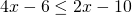Solution

2. Solve: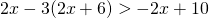Solution

3. Solve: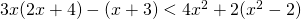Solution

4. Solve: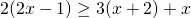Solution

5. Solve: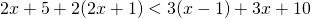Solution

6. Solve: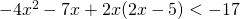Solution

7. Solve: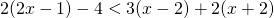Solution

8. For which value(s) of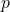does the following inequality have no solution: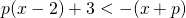Solution

9. Solve: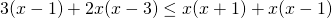Solution

10. Solve: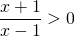Solution

0